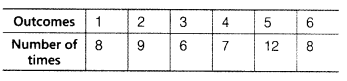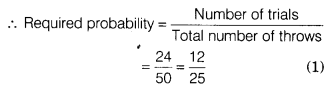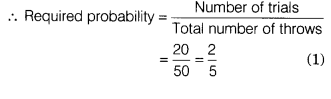# In 50 throws of a die, the outcomes were noted as under

In 50 throws of a die, the outcomes were noted as underA die is thrown at random. What is the probability of getting
(i) even number?
(ii) odd number?
(iii) greater than 4?

Given, total number of throws = 50
(i) Number of trials getting an even number
= 9+7+8 = 24Number of trial getting an odd number
= 8+6+12=26Number of trial getting the number greater than 4
= 12+8 = 20